Updating search results...

# 25 Results

View
Selected filters:
• Annenberg LearnerRating
0.0 stars
Overview:

An interactive resource on annotating and close textual reading from Solomon Northup's Twelve Years a Slave.

Subject:
English Language Arts
Material Type:
Interactive
Provider:
Annenberg Learner
Author:
Annenberg Learner
02/26/2019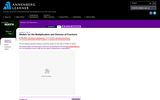Rating
0.0 stars
Overview:

Students will use area models to multiply and reduce fractions.

Subject:
Mathematics
Material Type:
Activity/Lab
Provider:
Annenberg Learner
Author:
Annenberg Learner
02/26/2019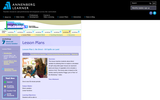Rating
3.0 stars
Overview:

This lesson teaches students about direct variation by allowing them to explore a simulated oil spill using toilet paper tissues (to represent land) and drops of vegetable oil (to simulate a volume of oil).

Subject:
Mathematics
Math 1
Material Type:
Lesson Plan
Provider:
Annenberg Learner
Author:
Annenberg Learner
02/26/2019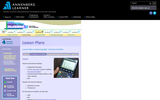Rating
5.0 stars
Overview:

Students explore quadratic functions by using a motion detector known as a Calculator Based Ranger (CBR) to examine the heights of the different bounces of a ball. Students will represent each bounce with a quadratic function of the form y = a (x - h)^2 + k. This lesson plan is based on the activity Tremain Nelson uses in the video for Part II of this workshop.

Subject:
Math 1
Math 2
Math 3
Material Type:
Lesson Plan
Provider:
Annenberg Learner
Author:
Annenberg Learner
02/26/2019Rating
5.0 stars
Overview:

Students use blocks to build a design from a written description. Try various descriptions to achieve the correct design.

Subject:
Mathematics
Material Type:
Activity/Lab
Provider:
Annenberg Learner
Author:
Annenberg Learner
02/26/2019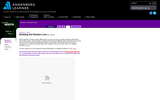Rating
0.0 stars
Overview:

Students classify the different types of numbers we use, and learn how numbers and operations relate to one another. They start with counting numbers and then add integers, rationals, algebraic, and, finally, real numbers to the line.

Subject:
Mathematics
Material Type:
Activity/Lab
Provider:
Annenberg Learner
Author:
Annenberg Learner
02/26/2019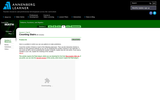Rating
3.0 stars
Overview:

Students build staircases out of square blocks. They look for patterns and come up with a rule or formula to predict the number of blocks in any staircase.

Subject:
Math 1
Math 3
Material Type:
Activity/Lab
Provider:
Annenberg Learner
Author:
Annenberg Learner
02/26/2019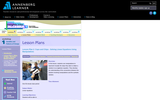Rating
0.0 stars
Overview:

Students use manipulatives to represent visually the steps they take to obtain a solution to an algebraic equation. They develop an understanding of the connections between the solution involving manipulatives and the symbolic solution.

Subject:
Math 1
Material Type:
Activity/Lab
Lesson Plan
Provider:
Annenberg Learner
Author:
Annenberg Learner
02/26/2019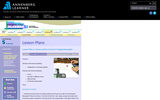Rating
0.0 stars
Overview:

Students learn about basic recursion by exploring patterns in the data they generate from two simple probability-based experiments.

Subject:
Math 1
Math 2
Math 3
Material Type:
Lesson Plan
Provider:
Annenberg Learner
Author:
Annenberg Learner
02/26/2019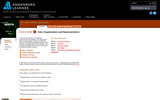Rating
0.0 stars
Overview:

Students collect data on the number of raisins in 17 boxes of raisins. They will use the data to analyze data and interprete results, answer a series of questions about variation, and construct data in plots.

Subject:
Mathematics
Material Type:
Activity/Lab
Provider:
Annenberg Learner
Author:
Annenberg Learner
02/26/2019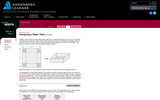Rating
0.0 stars
Overview:

Students Imagine constructing an open-topped water tank from a square metal sheet. They investigate the relationship between the maximum volume of the tank and the size of the squares cut from the corners, build models and collect data.

Subject:
Mathematics
Material Type:
Activity/Lab
Provider:
Annenberg Learner
Author:
Annenberg Learner
02/26/2019Educational Use
Rating
0.0 stars
Overview:

This video demonstrates the difference between heat and temperature and examines how both are defined in terms of particles. It illustrates the transfer of heat energy and uses a particle model to explain a number of everyday phenomena, including why things expand when they are heated to the role that temperature plays in changes of states of matter.

Subject:
Science
Material Type:
Demonstration
Provider:
Annenberg Learner
Author:
Annenberg Learner, etal
04/04/2014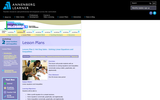Rating
4.0 stars
Overview:

This lesson will provide students with an introduction to solving equations and inequalities numerically (using a table), graphically, and algebraically.

Subject:
Math 1
Math 3
Material Type:
Activity/Lab
Lesson Plan
Provider:
Annenberg Learner
Author:
Annenberg Learner
02/26/2019Rating
0.0 stars
Overview:

Subject:
English Language Arts
Material Type:
Interactive
Provider:
Annenberg Learner
Author:
Annenberg Learner
02/26/2019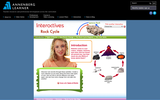Rating
0.0 stars
Overview:

Students can read short articles about rock types, the rock cycle, and identifying rocks. Students can collect rocks in a virtual rock collection and learn about each one. A short quiz is provided at the end for students to test their own knowledge.

Subject:
Science
Material Type:
Interactive
Provider:
Annenberg Learner
Author:
Annenberg Learner
02/26/2019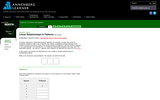Rating
3.0 stars
Overview:

Students explore the dependence of one variable on another and develop a formula describing the number of toothpicks as a function of the number of squares.

Subject:
Mathematics
Math 1
Material Type:
Lesson Plan
Provider:
Annenberg Learner
Author:
Annenberg Learner
02/26/2019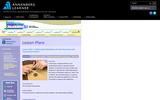Rating
3.0 stars
Overview:

Students will learn to use mathematical models to represent real life situations. In particular, they will use tables and equations to represent the relationship between the number of revolutions made by a "driver" and a "follower" (two connected gears in a system), and they will explain the significance of the radii of the gears in regard to this relationship.

Subject:
Math 1
Math 3
Material Type:
Lesson Plan
Provider:
Annenberg Learner
Author:
Annenberg Learner
02/26/2019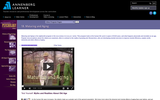Rating
0.0 stars
Overview:

Students will look at the human life cycle in spans of 20-25 years, and what happens physically and mentally as we age. Popular misconceptions about the eldery are examined, often in contrast to the reality of growing old.

Subject:
Psychology
Material Type:
Lesson
Provider:
Annenberg Learner
Author:
Annenberg Learner
02/26/2019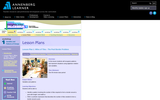Rating
0.0 stars
Overview:

Students will recognize patterns and represent situations using algebraic notation and variables.

Subject:
Mathematics
Math 3
Material Type:
Lesson Plan
Provider:
Annenberg Learner
Author:
Annenberg Learner
02/26/2019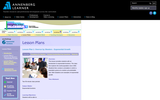Rating
4.0 stars
Overview:

This lesson provides students with an introduction to exponential functions. The class first explores the world population since 1650. Students then conduct a simulation in which a population grows at a random yet predictable rate. Both situations are examples of exponential growth.

Subject:
Math 1
Math 2
Math 3
Material Type:
Activity/Lab
Lesson Plan
Provider:
Annenberg Learner
Author:
Annenberg Learner